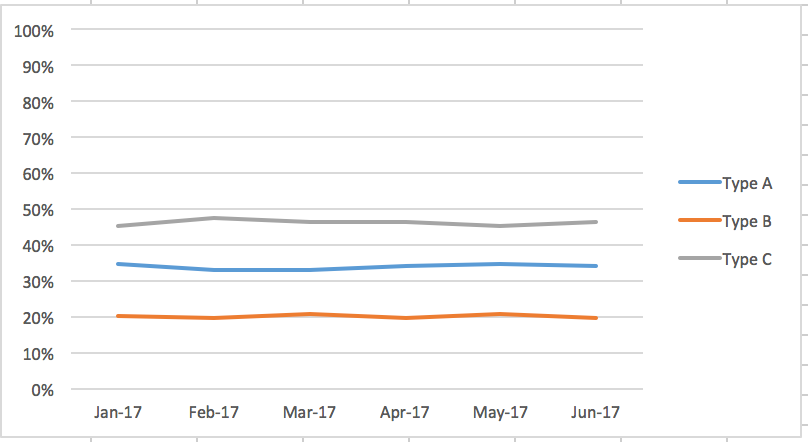# Line Percent of Total Chart

I'm struggling trying to figure out if Domo can handle creating a line chart that calculates the percent of total for the value, then show those values as percentages on a line chart. I've been searching around forever and have tried using %_PERCENT_OF_TOTAL as a data value label, but the problem there is that doesn't change the Y axis scale, it still shows the count as the scale, not 0-100%. By the title of property, I'd imagine that makes sense since it is just configuring the data labels.

My domo dataset columns are quite simple, just Date, Type (A,B,C) and Count. I can figure out how to do this quite simply in Excel, but not seeing an easy way to do this currently in Domo.

I've attached a quick sample image of what I'm trying to accomplish. Is this even possible in Domo?•CoachYou should be able to accomplish this with a beast mode calculation:

You will need to make a separate calculation for each type.

Type A:

sum(case when `Type` = 'Type A' then `Count` end) / sum(`Count`)

Type B:

sum(case when `Type` = 'Type B' then `Count` end) / sum(`Count`)

Type C:

sum(case when `Type` = 'Type C' then `Count` end) / sum(`Count`)

You should then be able to use the Line Chart.  Just put `Type A` for Y-axis, and `Type B` and `Type C` in as SERIES.

“There is a superhero in all of us, we just need the courage to put on the cape.” -Superman
• This original question was posed 4 years ago.

Is there a more modern way to accomplish this without creating a unknowable number of beast-mode calculations?

•Coachwindow function.

sum(amount) / sum(sum(amount))